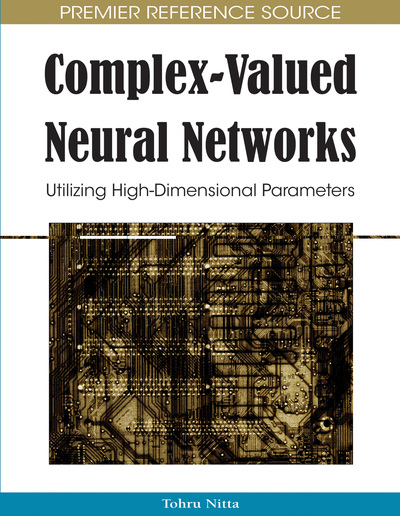# Models of Complex-Valued Hopfield-Type Neural Networks and Their Dynamics

Yasuaki Kuroe
DOI: 10.4018/978-1-60566-214-5.ch006
OnDemand:
(Individual Chapters)
Available
\$37.50
No Current Special Offers

## Abstract

This chapter presents models of fully connected complex-valued neural networks which are complex-valued extension of Hopfield-type neural networks and discusses methods to study their dynamics. In particular the authors investigate existence conditions of energy functions for complex-valued Hopfield-type neural networks. Emphasized are the properties of the activation functions which assure the existence of an energy function for the networks. As an application of the energy function, qualitative analysis of the network by utilizing the energy function is shown and a synthesis method of complex-valued associative memories is discussed.
Chapter Preview
Top

## Introduction

In recent years, there have been increasing research interests of artificial neural networks and many efforts have been made on applications of neural networks to various fields. As applications of the neural networks spread more widely, developing neural network models which can directly deal with complex numbers is desired in various fields. Several models of complex-valued neural networks have been proposed and their abilities of information processing have been investigated.

The purpose of this chapter is to present models of fully connected complex-valued neural networks which are complex-valued extension of Hopfield-type neural networks and to discuss methods to investigate their dynamics. In particular, we investigate existence conditions of energy functions and propose a function as an energy function for the complex-valued neural networks. In the complex region there are several possibilities in choosing an activation function because of a variety of complex functions. Based on the existence condition we investigate the properties of the complex functions which assure the existence of an energy function and discuss about how to find them. Several classes of complex functions which are widely used as activation functions in the models of complex-valued neural networks proposed so far are considered.

Once energy functions are constructed for neural networks, they are expected to be applied to various problems of various fields such as qualitative analysis of the neural networks, synthesis of associative memories and several optimization problems, similar to the real-valued ones. As applications, this chapter presents qualitative analysis of dynamics of the complex-valued neural networks by using the energy function. Furthermore a synthesis method of complex-valued associative memories by utilizing the analysis results is discussed.

## Complete Chapter List

Search this Book:
Reset